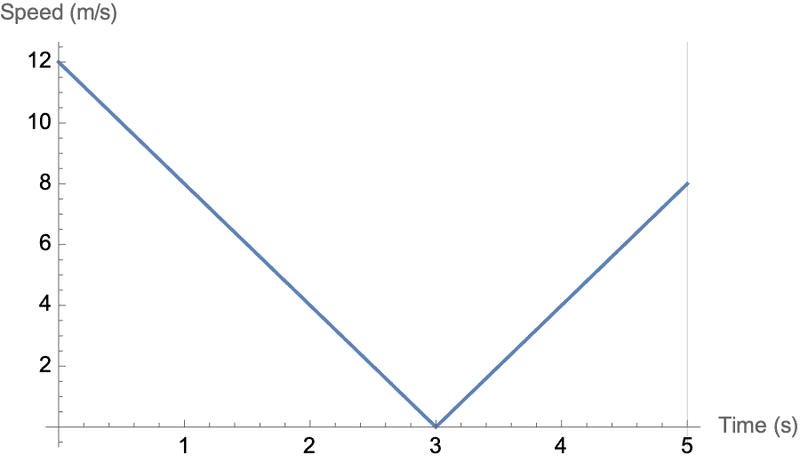# Displacement of particle

• rudransh verma

#### rudransh verma

Gold Member
Homework Statement
The displacement of a particle moving in straight line is given by ##s=6+12t-2t^2##. The distance covered by particle in first 5sec. Units are in meters and sec.
Relevant Equations
##v=\frac{ds}{dt}##
I calculated v=0 at t=3. s(3) =24 m. s(5)=16 m. So reverse distance that the particle travelled=24-16=8 m. So total distance =24+8=32 m.

But s=6 at t=0. Did you take this into account?

•Delta2
But s=6 at t=0. Did you take this into account?
O yes! I thought the position is origin at t=0. So it will be 32-6=26 meters. Thanks. Is there any other way to do it ?

Well, you could take the velocity, which is v = ds/dt = 12 - 4t, and then find $\int_0^5 |v| dt = 26$.

Is there any other way to do it ?
You can also plot speed vs. time and find the area under the curve (see below) which is easy in this case of two right triangles. This is the geometric equivalent of what @phyzguy suggested in post #4.•rudransh verma, SammyS and Delta2
You can also plot speed vs. time and find the area under the curve
Ok! By the way why we use area under the curve thing.
Is it because finding area under the curve (integral) give the distance covered and we use the same concept of finding the area except it’s easy here because there are two triangles?

Last edited:
Ok! By the way why we use area under the curve thing.
Is it because finding area under the curve (integral) give the distance covered and we use the same concept of finding the area except it’s easy here because there are two triangles?
Yes.